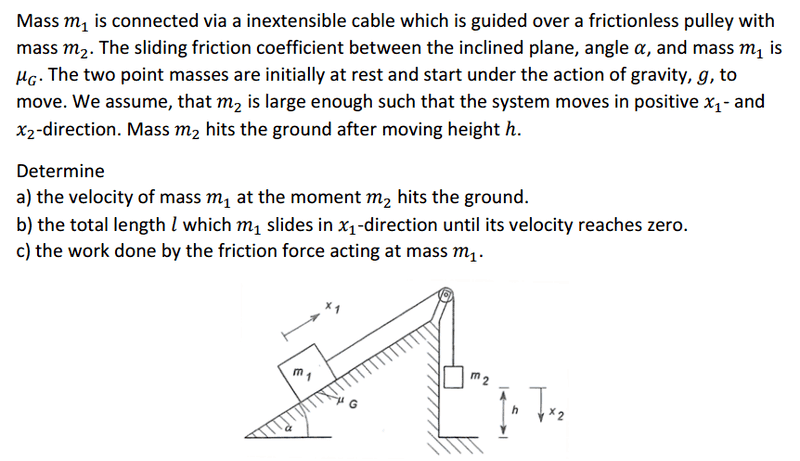# Calculate the velocity of a mass

Member warned about not using the homework template
Hi
I have this question to do :I want to calculate the velocity ... I did like this:

m2 * a = m2 * g - T
m1 * a = T - μ*m1*g*sin α
combine them
m1 * a = -m2*a + m2*g - μ*m1*g*sin α

Now what should I do ?
thanks

## Answers and Replies

m2 * a = m2 * g - T
m1 * a = T - μ*m1*g*sin α
combine them
m1 * a = -m2*a + m2*g - μ*m1*g*sin α

Now what should I do ?
thanks

why you ask? the vertical mass can cover only a finite distance ..... so the inclined plane -mass should cover the same distance as string/cable is taut..... then from above equations you can calculate the acceleration so you know speed... well i may be wrong... but one can try.

why you ask? the vertical mass can cover only a finite distance ..... so the inclined plane -mass should cover the same distance as string/cable is taut..... then from above equations you can calculate the acceleration so you know speed... well i may be wrong... but one can try.

ok the acceleration is

a=(m2*g - μ*m1*g*sin α)/(m1+m2)
and v=a/t
but I do not have t (time)

ok the acceleration is

a=(m2*g - μ*m1*g*sin α)/(m1+m2)
and v=a/t
but I do not have t (time)

are you correct in writing above equation for 'a' and v ? think...
connected systems have same displacements, velocity-magnitude and accelerations. v=a/t is not correct dimensionally - as it is accelerated motion with initial vel=0 one can use v= sqrt(2.acceleration. distance covered)

Ray Vickson
Science Advisor
Homework Helper
Dearly Missed
ok the acceleration is

a=(m2*g - μ*m1*g*sin α)/(m1+m2)
and v=a/t
but I do not have t (time)

If the acceleration ##a## is constant, you have a simple problem of an object falling with constant acceleration. The acceleration happens to be different from ##g## in this case, but that does not matter; the equations are exactly the same as those you must have seen already in previous courses; if not, you can solve the differential equation for the motion., to get ##x(t)##.

ok the acceleration is

a=(m2*g - μ*m1*g*sin α)/(m1+m2)
and v=a/t
but I do not have t (time)

In that case I believe that you could use the kinematic equation V2 = V02+2ax
Assuming that the acceleration is constant which it should be since it is caused by gravity in this case